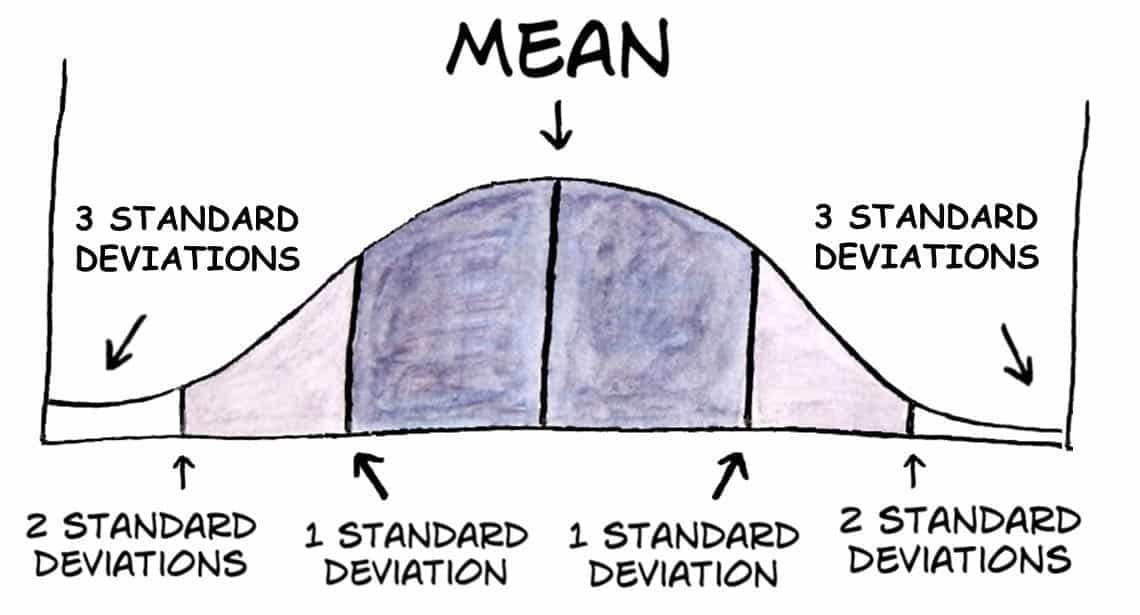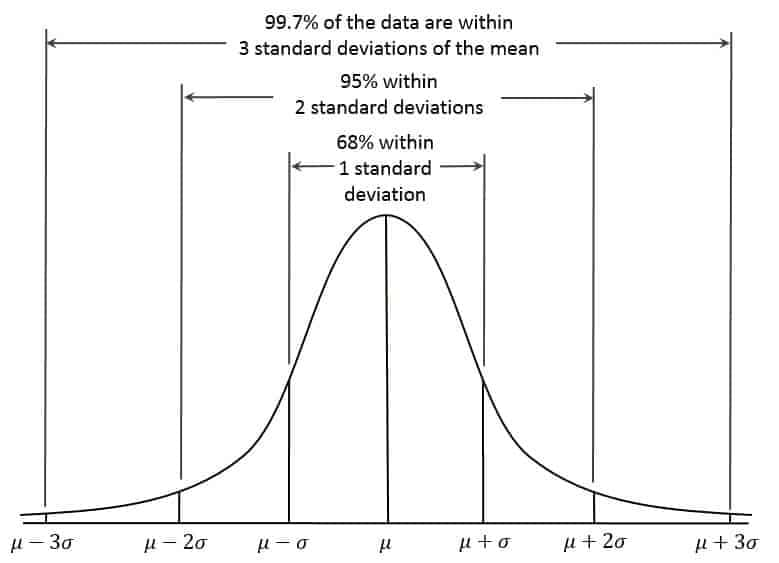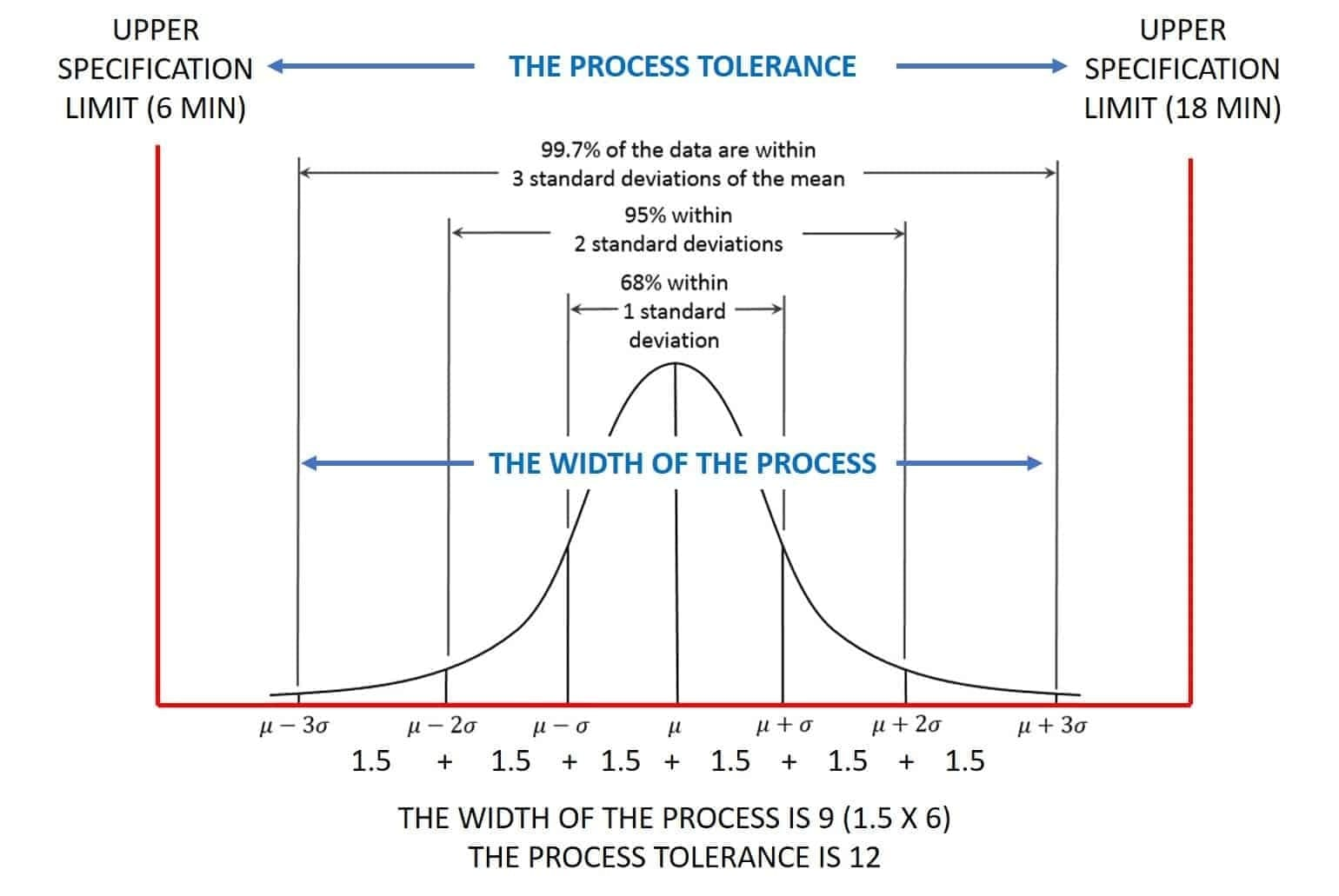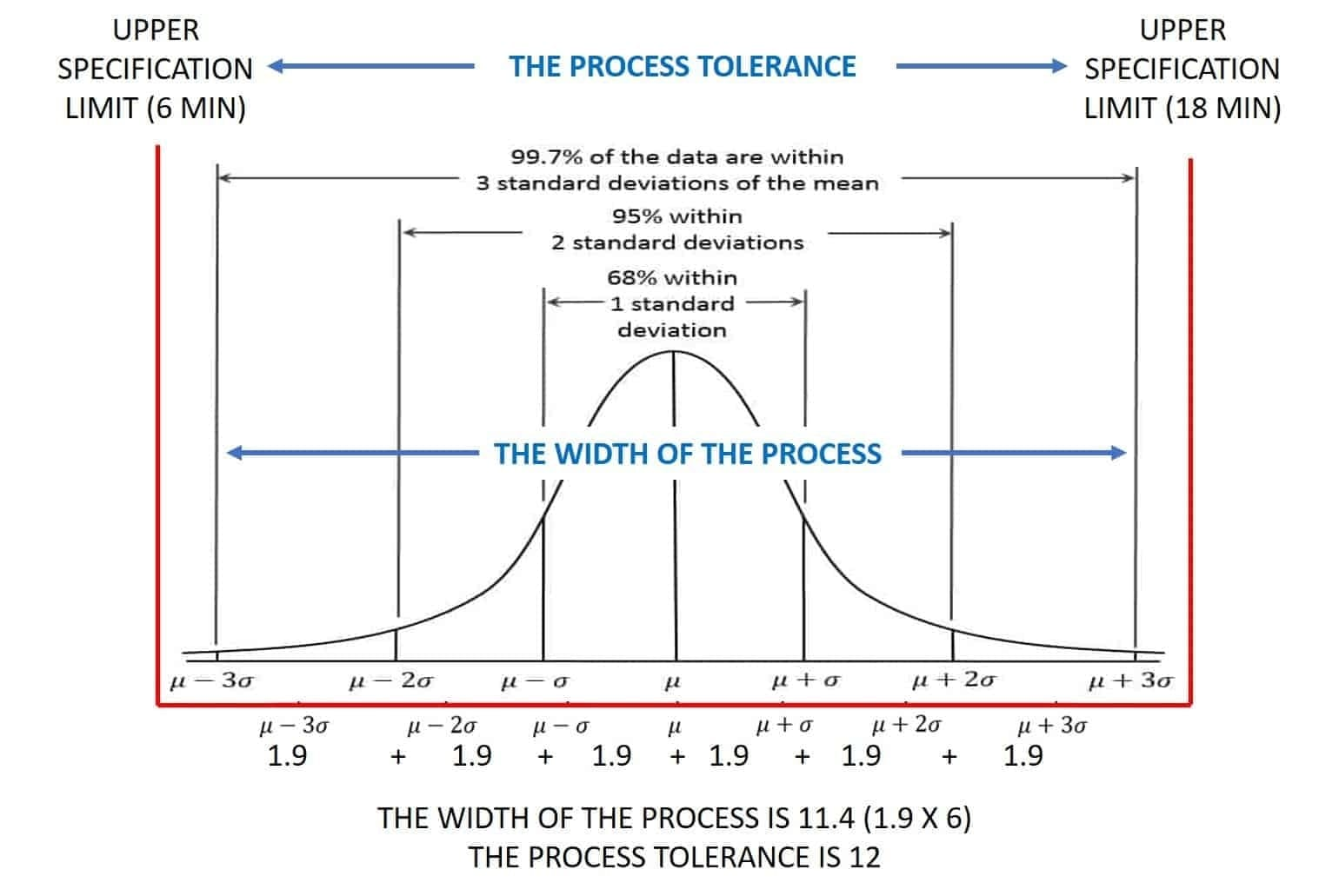Select Page## Using “Standard Deviation” as a Measure of Data Spread or Dispersion

A measure of dispersion tells you the spread of the data. This is important to know the spread of your data when describing your data set. Most describe a set of data by using only the mean or median leaving out a description of the spread.

There are two main measures of spread or dispersion:

• Range
• Standard Deviation

### In this article we will discuss the Standard Deviation of the Distribution

The standard deviation (s) is the most common measure of dispersion. Standard deviation tells you how spread out or dispersed the data is in the data set. It is a measure of how far each observed value in the data set is from the mean. In any distribution, theoretically 99.73% of values will be within +-3 standard deviations of the mean.### Why is Standard Deviation Important in Lean Six Sigma?

Let’s look at an example. Let’s say I owned a company that produced widgets as a sub-component for other parts. Every time a widget is produced, a system records the time it took from start to finish of the widget. The requirements for the time to complete a widget is no less than 6 minutes and no more than 18 minutes.

In the below example, we calculate the standard deviation as 1.5 minutes from a sample of 60 widgets.You can see from this process that it can perform within the specification limits or the process tolerance.

What happens if the the standard deviation increases? Let’s see:As the standard deviation of the process increases (which means that variation in the process is increasing), the width of the process grows  bringing it closer to exceeding the process tolerance.

These illustrations show that knowing the standard deviation and tolerance of a process can show the performance of the process. Knowing the performance of a process is critical in a Lean Six Sigma project.LEARN THE 10 SIMPLE STEPS THAT WE TEACH LEAN SIX SIGMA GREEN BELTS TO GET AMAZING RESULTS FROM THEIR PROJECTS.